Question

# determine the pH of a solution containing 15.00mL of 0.1163 M LiOH with 42.92mL of 0.05643M...

determine the pH of a solution containing 15.00mL of 0.1163 M LiOH with 42.92mL of 0.05643M HNO3

LiOH + HNO3 -----------> LiNO3 + H2O

moles of LiOH = 0.1163 x 15/1000 = 0.00174

moles of HNO3 = 0.05643 x 42.92 / 1000 = 0.002422

0.002422 - 0.00174 = 0.000682 moles HNO3 left

[HNO3] = 0.000682 / 0.05792

[HNO3] = 0.012 M

HNO3 is strong acid so [HNO3] = [H+] = 0.012 M

pH = - log [H+]

pH = - log [0.012]

pH = 1.92

#### Earn Coins

Coins can be redeemed for fabulous gifts.

Similar Homework Help Questions
• ### Calculate the ph Calculate the pH in the following cases: 1. A solution containing 0.250 M...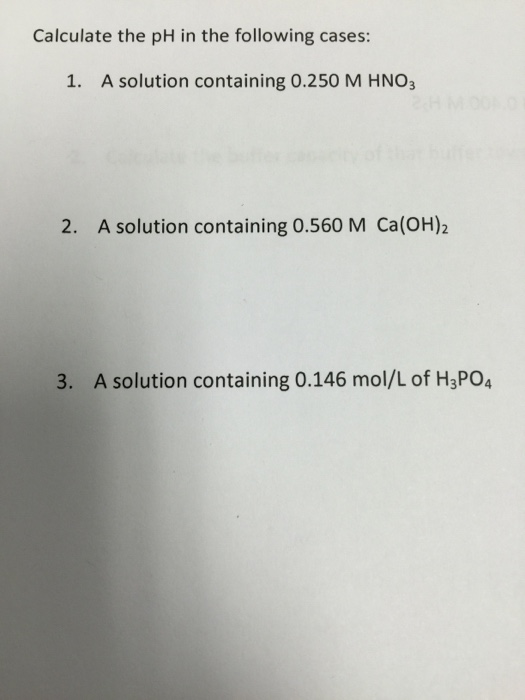Calculate the ph Calculate the pH in the following cases: 1. A solution containing 0.250 M HNO3 2. A solution containing 0.560 M Ca(OH)2 3. A solution containing 0.146 mol/L of H3PO4

• ### calculate the ph of the following solution: 15.00ml of 0.1400 M Na Oh has been added...

calculate the ph of the following solution: 15.00ml of 0.1400 M Na Oh has been added to 25.00 00ml of a buffer which is 0.2500 M HAc and .2000 M NaAc. Ka HAc= 1.8x10^-5

• ### Question 9 of 11 > Determine the pH of a solution containing 0.050 M NaOH and...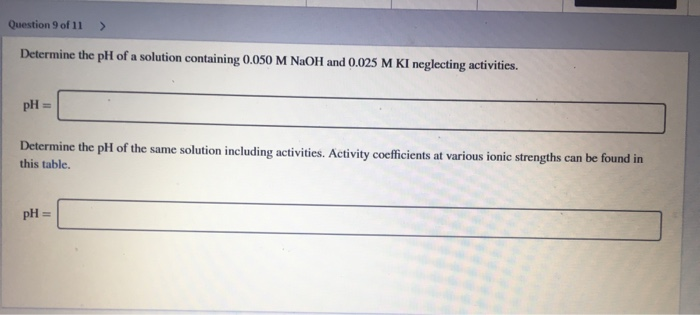Question 9 of 11 > Determine the pH of a solution containing 0.050 M NaOH and 0.025 M KI neglecting activities. pH = Determine the pH of the same solution including activities. Activity coefficients at various ionic strengths can be found in this table. pH =

• ### A 74.0 mL sample of 0.0400 M HNO3 is titrated with 0.0800 M LiOH solution. Calculate...

A 74.0 mL sample of 0.0400 M HNO3 is titrated with 0.0800 M LiOH solution. Calculate the pH after the following volumes of base have been added. (a) 11.8 mL pH = (b) 36.3 mL pH = (c) 37.0 mL pH = (d) 38.9 mL pH = (e) 59.2 mL pH =

• ### A 400.0 mL sample of 0.18 M HClO4 is titrated with 0.27 M LiOH. Determine the...

A 400.0 mL sample of 0.18 M HClO4 is titrated with 0.27 M LiOH. Determine the pH of the solution after the addition of 200.0 mL of LiOH. 0.68 2.35 1.52 3.22 12.48

• ### Form 1 29. Determine the pH of a 0.741 M LiOH solution at 25°C. A) 0.130...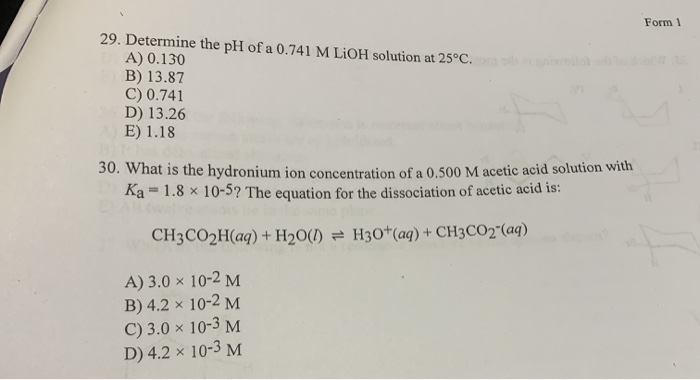Form 1 29. Determine the pH of a 0.741 M LiOH solution at 25°C. A) 0.130 B) 13.87 C) 0.741 D) 13.26 E) 1.18 30. What is the hydronium ion concentration of a 0.500 M acetic acid solu Ka = 1.8 x 10-5? The equation for the dissociation of acetic acid is: centration of a 0.500 M acetic acid solution with CH3CO2H(aq) + H2O(1) = H30+(aq) + CH3CO2"(aq) A) 3.0 x 10-2 M B) 4.2 x 10-2 M C) 3.0...

• ### What will happen to pH of 0.5L of buffer solution containing 0.4 M NHz and 0.8M...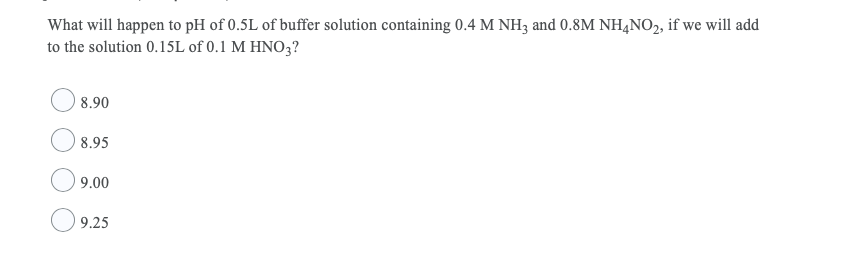What will happen to pH of 0.5L of buffer solution containing 0.4 M NHz and 0.8M NH4NO2, if we will add to the solution 0.15L of 0.1 M HNO3? 8.90 8.95 9.00 9.25

• ### A 900.0 mL sample of 0.18 M HCIO4 is titrated with 0.27 M LIOH, Determine the...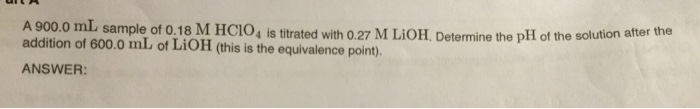A 900.0 mL sample of 0.18 M HCIO4 is titrated with 0.27 M LIOH, Determine the pH of the solution after the addition of 600.0 mL of LIOH (this is the equivalence point). ANSWER: 0.97 7.00 11.24 13.03 2.76

• ### Determine the pH of the following solutions: 5.9 x 10-M H2SO4 0.0014 M LiOH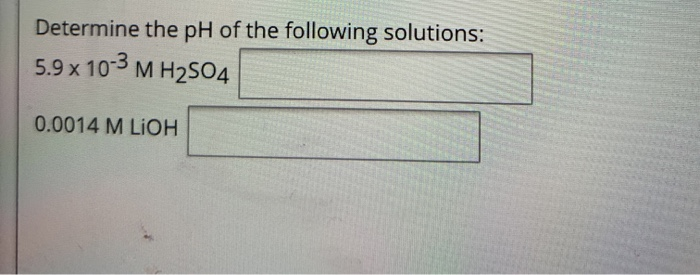Determine the pH of the following solutions: 5.9 x 10-M H2SO4 0.0014 M LiOH

• ### Determine the pH of a solution containing 0.050 M NaOH and 0.025 M KI neglecting activities....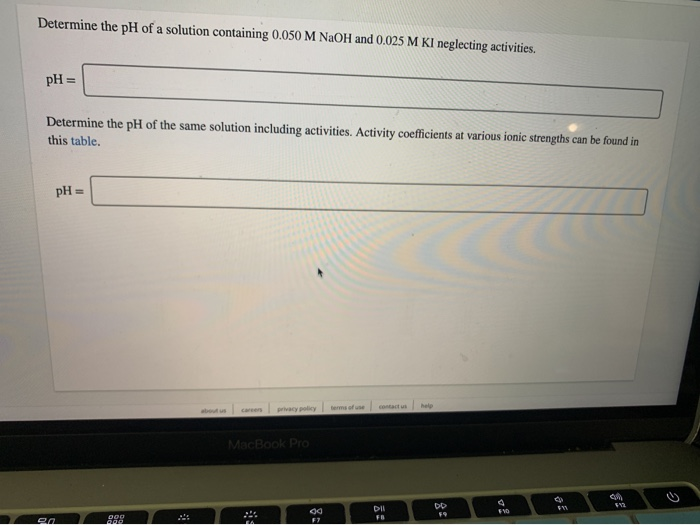Determine the pH of a solution containing 0.050 M NaOH and 0.025 M KI neglecting activities. pH= Determine the pH of the same solution including activities. Activity coefficients at various ionic strengths can be found in this table, pH= contact u help terms of use privacy policy about us careers MacBook Pro DIl FB en F7 8